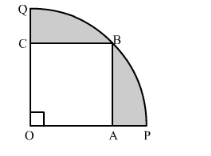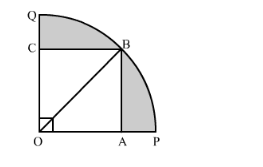# In the following figure a square OABC is inscribed in a quadrant OPBQ of a circle.

Question:

In the following figure a square OABC is inscribed in a quadrant OPBQ of a circle. If OA = 21 cm, find the area of the shaded region.Solution:

Construction: Join OBIn right triangle AOB

$\mathrm{OB}^{2}=\mathrm{OA}^{2}+\mathrm{AB}^{2}$

$=21^{2}+21^{2}$

$=441+441$

$=882$

$\therefore \mathrm{OB}^{2}=882$

Area of the shaded region = Area of quadrant OPBQ − Area of Square OABC

$=\frac{1}{4} \pi(\mathrm{OB})^{2}-(\mathrm{OA})^{2}$

$=\frac{1}{4} \times \frac{22}{7} \times 882-441$

$=693-441$

$=252 \mathrm{~cm}^{2}$

Hence, the area of the shaded region is 252 cm2.